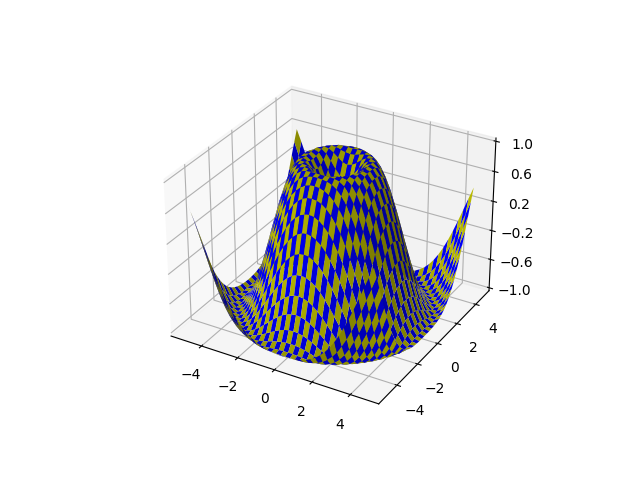# 3D surface (checkerboard)#

Demonstrates plotting a 3D surface colored in a checkerboard pattern.import matplotlib.pyplot as plt
from matplotlib.ticker import LinearLocator
import numpy as np

# Make data.
X = np.arange(-5, 5, 0.25)
xlen = len(X)
Y = np.arange(-5, 5, 0.25)
ylen = len(Y)
X, Y = np.meshgrid(X, Y)
R = np.sqrt(X**2 + Y**2)
Z = np.sin(R)

# Create an empty array of strings with the same shape as the meshgrid, and
# populate it with two colors in a checkerboard pattern.
colortuple = ('y', 'b')
colors = np.empty(X.shape, dtype=str)
for y in range(ylen):
for x in range(xlen):
colors[y, x] = colortuple[(x + y) % len(colortuple)]

# Plot the surface with face colors taken from the array we made.
surf = ax.plot_surface(X, Y, Z, facecolors=colors, linewidth=0)

# Customize the z axis.
ax.set_zlim(-1, 1)
ax.zaxis.set_major_locator(LinearLocator(6))

plt.show()


Keywords: matplotlib code example, codex, python plot, pyplot Gallery generated by Sphinx-Gallery